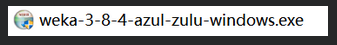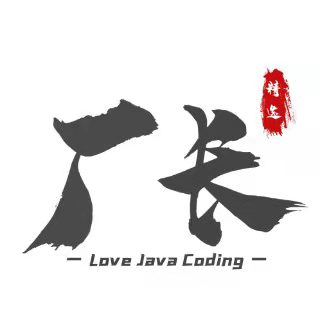# 用 WEKA 进行数据挖掘，第 3 部分 最近邻和服务器端库

+关注继续查看

## 最近邻

### 最近邻背后的数学理论

##### 清单 1. 最近邻的数学理论
Customer     Age     Income     Purchased Product

1            45       46k       Book

2            39       100k      TV

3            35       38k       DVD

4            69       150k      Car Cover

5            58       51k       ???

Step 1:  Determine Distance Formula

Distance = SQRT( ((58 - Age)/(69-35))^2) + ((51000 - Income)/(150000-38000))^2 )

Step 2:  Calculate the Score

Customer     Score     Purchased Product

1            .385         Book

2            .710         TV

3            .686         DVD

4            .941         Car Cover

5            0.0          ???

## 在服务器上使用 WEKA

: 我最好提前告诫您 WEKA API 有时很难导航。首要的是要复核所用的 WEKA 的版本和 API 的版本。此 API 在不同的发布版间变化会很大，以至于代码可能会完全不同。而且，即便此 API 完备，却没有什么非常好的例子可以帮助我们开始（当然了，这也是为什么您在阅读本文的原因）。我使用的是 WEKA V3.6。

##### 清单 4. 将数据载入 WEKA
// Define each attribute (or column), and give it a numerical column number

// Likely, a better design wouldn't require the column number, but

// would instead get it from the index in the container

Attribute a1 = new Attribute("houseSize", 0);

Attribute a2 = new Attribute("lotSize", 1);

Attribute a3 = new Attribute("bedrooms", 2);

Attribute a4 = new Attribute("granite", 3);

Attribute a5 = new Attribute("bathroom", 4);

Attribute a6 = new Attribute("sellingPrice", 5);

// Each element must be added to a FastVector, a custom

// container used in this version of Weka.

// Later versions of Weka corrected this mistake by only

// using an ArrayList

FastVector attrs = new FastVector();

attrs.addElement(a1);

attrs.addElement(a2);

attrs.addElement(a3);

attrs.addElement(a4);

attrs.addElement(a5);

attrs.addElement(a6);

// Each data instance needs to create an Instance class

// The constructor requires the number of columns that

// will be defined.  In this case, this is a good design,

// since you can pass in empty values where they exist.

Instance i1 = new Instance(6);

i1.setValue(a1, 3529);

i1.setValue(a2, 9191);

i1.setValue(a3, 6);

i1.setValue(a4, 0);

i1.setValue(a5, 0);

i1.setValue(a6, 205000);

....

// Each Instance has to be added to a larger container, the

// Instances class.  In the constructor for this class, you

// must give it a name, pass along the Attributes that

// are used in the data set, and the number of

// Instance objects to be added.  Again, probably not ideal design

// to require the number of objects to be added in the constructor,

// especially since you can specify 0 here, and then add Instance

// objects, and it will return the correct value later (so in

// other words, you should just pass in '0' here)

Instances dataset = new Instances("housePrices", attrs, 7);

dataset.add(i1);

dataset.add(i2);

dataset.add(i3);

dataset.add(i4);

dataset.add(i5);

dataset.add(i6);

dataset.add(i7);

// In the Instances class, we need to set the column that is

// the output (aka the dependent variable).  You should remember

// that some data mining methods are used to predict an output

// variable, and regression is one of them.

dataset.setClassIndex(dataset.numAttributes() - 1);


##### 清单 5. 在 WEKA 内创建回归模型
// Create the LinearRegression model, which is the data mining

// model we're using in this example

LinearRegression linearRegression = new LinearRegression();

// This method does the "magic", and will compute the regression

// model.  It takes the entire dataset we've defined to this point

// When this method completes, all our "data mining" will be complete

// and it is up to you to get information from the results

linearRegression.buildClassifier(dataset);

// We are most interested in the computed coefficients in our model,

// since those will be used to compute the output values from an

// unknown data instance.

double[] coef = linearRegression.coefficients();

// Using the values from my house (from the first article), we

// plug in the values and multiply them by the coefficients

// that the regression model created.  Note that we skipped

// coefficient as that is 0, because it was the output

// variable from our training data

double myHouseValue = (coef * 3198) +

(coef * 9669) +

(coef * 5) +

(coef * 3) +

(coef * 1) +

coef;

System.out.println(myHouseValue);

// outputs 219328.35717359098

// which matches the output from the earlier article

## 结束语

### 下载资源856 01105 01739 01152 02222 01087 0《R语言与数据挖掘最佳实践和经典案例》—— 导读
1371 0《R语言与数据挖掘最佳实践和经典案例》—— 3.5　将图表保存到文件中
1170 0
hiekay
java 数据分析 数据可视化 大数据1103283144528108618

【算法实战】7. 集成方法-随机森林576265